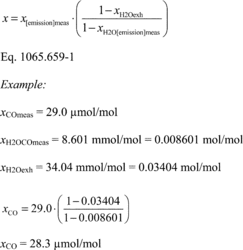# Title 40

## SECTION 1065.659

### 1065.659 Removed water correction.

§ 1065.659 Removed water correction.

(a) If you remove water upstream of a concentration measurement, x, correct for the removed water. Perform this correction based on the amount of water at the concentration measurement, xH2O[emission]meas, and at the flow meter, xH2Oexh, whose flow is used to determine the mass emission rate or total mass over a test interval. For continuous analyzers downstream of a sample dryer for transient and ramped-modal cycles, you must apply this correction on a continuous basis over the test interval, even if you use one of the options in § 1065.145(e)(2) that results in a constant value for xH2O[emission]meas because xH2Oexh varies over the test interval. For batch analyzers, determine the flow-weighted average based on the continuous xH2Oexh values determined as described in paragraph (c) of this section. For batch analyzers, you may determine the flow-weighted average xH2Oexh based on a single value of xH2Oexh determined as described in paragraphs (c)(2) and (3) of this section, using flow-weighted average or batch concentration inputs.

(b) Determine the amount of water remaining downstream of a sample dryer and at the concentration measurement using one of the methods described in § 1065.145(e)(2). If you use a sample dryer upstream of an analyzer and if the calculated amount of water remaining downstream of the sample dryer and at the concentration measurement, xH2O[emission]meas, is higher than the amount of water at the flow meter, xH2Oexh, set xH2O[emission]meas equal to xH2Oexh. If you use a sample dryer upstream of storage media, you must be able to demonstrate that the sample dryer is removing water continuously (i.e., xH2Oexh is higher than xH2O[emission]meas throughout the test interval).

(c) For a concentration measurement where you did not remove water, you may set xH2O[emission]meas equal to xH2Oexh. You may determine the amount of water at the flow meter, xH2Oexh, using any of the following methods:

(1) Measure the dewpoint and absolute pressure and calculate the amount of water as described in § 1065.645.

(2) If the measurement comes from raw exhaust, you may determine the amount of water based on intake-air humidity, plus a chemical balance of fuel, intake air, and exhaust as described in § 1065.655.

(3) If the measurement comes from diluted exhaust, you may determine the amount of water based on intake-air humidity, dilution air humidity, and a chemical balance of fuel, intake air, and exhaust as described in § 1065.655.

(d) Perform a removed water correction to the concentration measurement using the following equation:[73 FR 37335, June 30, 2008, as amended at 76 FR 57462, Sept. 15, 2011; 79 FR 23804, Apr. 28, 2014]